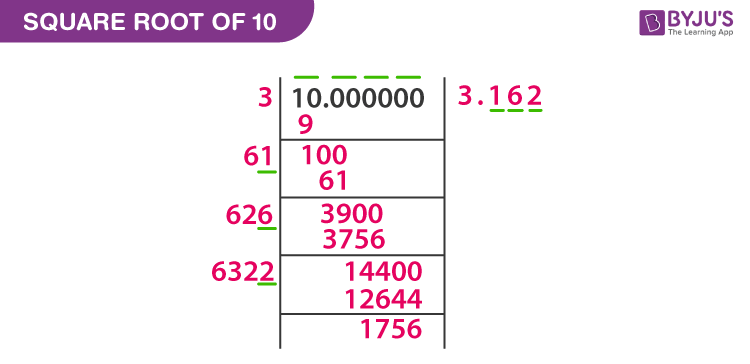# Square Root of 10

Square number or square of a number will be obtained when we multiply the number with the number itself. But there are different methods and representation available to find the square root of 10. Consider a few examples for the square numbers.

For example,

42 = 4 × 4 = 16, which represents the square of a number 4

112 = 11 × 11 = 121, which represents the square of a number 11

82 = 8 × 8 = 64, which represents the square of a number 8

So, in the above examples, 4, 10, and 8 are the square numbers. If we want to find the given number is a perfect square or not, then check the unit place of a number.

• The number is not a perfect square if the number ends with 2,3,7 and 8.
• The number is said to be a perfect square if the unit place of a number ends with 1,4,5,6 and 9.

## How to Find Square Root of 10?

Finding the square root of 10 is a bit complicated because the number…… because the number 10 is not a perfect square which has a number 0 at its unit place. Finding the square root of a number is the reverse process of squaring the number. We can find the square root of 10 using two methods. One method is to find the root values using unit places and another method is with the help of long division method. From the above examples, we can see number 16, 121, and 64 are the perfect squares, which have the unit place as 6, 1 and 4 respectively.

The symbol to denote the square root is ‘√’. This is also called as a radical symbol or radix. The number underneath the square root symbol is called radicand. The value of the square root is represented in radical form as well as in decimal form. Here we will discuss the square root of 10, where the number 10 is called the radicand.

## What is the square root of 10?

The radical of 10 or the square root of 10 is represented in the form of √10. We know that number 10 is an even number but it is not a prime number. Prime numbers have the property of having only two factors of a number such as 1 and the number itself. But as we know, 10 have four multiple factors, 1,2,5 and 10 itself, it is not a prime number and the factors are written as

1 × 10 = 10

2 × 5 = 10

5 × 2 = 10

10 × 1 =10

But when the question comes, how can we find out the square roots for the value of 10? First, write the factors of 10 as given below.

10 = 2 × 5

In the above expression, you can see that the square number is not available on the right-hand side. So, the square root of 10 can be written as;

$$\begin{array}{l}\sqrt{10} = \sqrt{2 × 5}\end{array}$$

We cannot take the square term out of the root, because it has no square terms and it is written as,

$$\begin{array}{l}\sqrt{10} = \sqrt{2}\sqrt{5}\end{array}$$

The above expression is the radical form of  √10. If we want to write it in decimal form, put the values of √2 and √5 which is approximately equal to 1.414 and 2.236, respectively. Hence,

$$\begin{array}{l}\sqrt{10} = 1.414 \times 2.236\end{array}$$
$$\begin{array}{l}\sqrt{10} = \pm 3 .162 \ \text{approximately.}\end{array}$$

## Square Root of 10 by Long Division Method

To find the square root of 10 approximately, long division method is used. Use the long division procedure to find the value as follows.Similarly, for the number like 10, which are not perfect squares use the same method to find the square root value. For example,12, 18, 20, 27, etc. are not perfect squares, as they give the square root value in radical form as well as in decimal form.

## Video Lessons on Square Roots

### Visualising square roots### Finding Square rootsTo find the square roots of numbers, download BYJU’S – The Learning App and learn the concepts with the help of interactive videos.

 Find some other square roots here Square Root Of 1 Square Root Of 2 Square Root Of 3 Square Root Of 4 Square Root Of 5 Square Root Of 6 Square Root Of 7 Square Root Of 8
Test your Knowledge on Square Root of 10